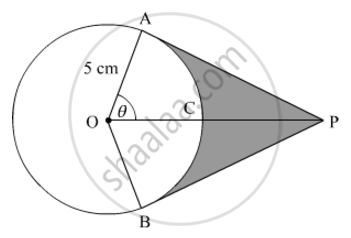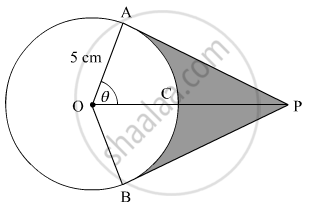Share

# An elastic belt is placed around the rim of a pulley of radius 5 cm. (Fig. 10) From one point C on the belt, the elastic belt is pulled directly away from the centre O of the pulley until it is at P, 10 cm from the point O. - CBSE Class 10 - Mathematics

ConceptProblems Based on Areas and Perimeter Or Circumference of Circle, Sector and Segment of a Circle

#### Question

An elastic belt is placed around the rim of a pulley of radius 5 cm. (Fig. 10) From one point C on the belt, the elastic belt is pulled directly away from the centre O of the pulley until it is at P, 10 cm from the point O. Find the length of the belt that is still in contact with the pulley. Also find the shaded area (use π = 3.14 and sqrt3=1.73)#### SolutionGiven:

OA = 5 cm

OP = 10 cm

We know that the tangent at any point of a circle is perpendicular to the radius through the point of contact.

Therefore, ∆OAP is a right-angled triangle.

OAP=90°

Now,

OP2 = OA2 + AP2

⇒ 102 = 52 + AP2

⇒ AP2 = 75

⇒ AP = 53sqrt3 cm

Also,

costheta=(OA)/(OP) = 5/10

=>costheta=1/2

θ=60°

Now,

AOP=BOP=60°  (OAPOBP)

AOB=120°

Length of the belt still in contact with the pulley = Circumference of the circle − Length of the arc ACB

=2xx3.14xx5-120^@/360^@xx2xx3.14xx5

=2xx3.14xx5xx(1-1/3)

=2xx3.14xx5xx2/3

=20.93 cm    (Approx.)

Now

Area of ∆OAP =

1/2xxAPxxOA=1/2xx5sqrt3xx5=(25sqrt3)/2 cm^2

Similarly,

Area of ∆OBP = (25sqrt3)/2cm^2

∴ Area of ∆OAP + Area of ∆OBP = 25sqrt3cm2=25×1.73=43.25 cm2

Area of sector OACB = 120^@/360^@xx3.14xx(5^2)=1/3xx3.14xx25=26.17cm^2

∴ Area of the shaded region = (Area of ∆OAP + Area of ∆OBP) − Area of the sector OACB

= 43.25 cm2 − 26.17 cm2

= 17.08 cm2    (Approx.)

Is there an error in this question or solution?

#### Video TutorialsVIEW ALL 

Solution An elastic belt is placed around the rim of a pulley of radius 5 cm. (Fig. 10) From one point C on the belt, the elastic belt is pulled directly away from the centre O of the pulley until it is at P, 10 cm from the point O. Concept: Problems Based on Areas and Perimeter Or Circumference of Circle, Sector and Segment of a Circle.
S